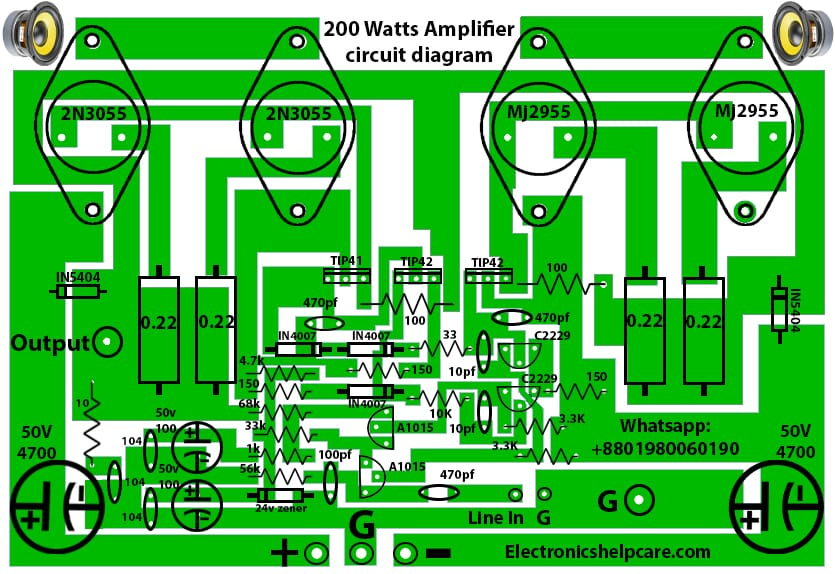# how to make 200 watts amplifier?

## This is 200 watts amplifier circuit diagram:

This is a 200 watts amplifier circuit diagram. here in this diagram, we can use 2n3055 and mj2955. 2n3055 is an NPN-positive transistor. and mj2955 is a PNP negative transistor.  2 transistors can make 100 watts. here used 4 transistors. so, it’s 200 watts circuit. here need dual voltage for this circuit. here in this circuit, we can use 35-0-35 voltage and 5 amperes.We have to use a good quality transformer. because watts depend on the good voltage. if we don’t use a good voltage then we can’t get good watts.

This is the back side of this circuit. we can make this circuit using this circuit diagram.This is a metal transistor circuit diagram. if we want a Silicon transistor circuit diagram. like 2sc5200 and 2sa1943. then please visit here 2sc5200 and 2sa1943 transistor amplifier circuit diagram  If we want please visit here.

Thanks a lot to be with us. If you want another post then please visit our website.

We have another post for you. like as repairing amplifier,